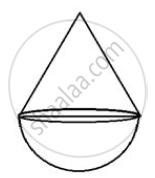Share

# A Cone of Height 15 Cm and Diameter 7 Cm is Mounted on a Hemisphere of Same Diameter. Determine the Volume of the Solid Thus Formed. - ICSE Class 10 - Mathematics

ConceptArea and Volume of Solids - Cone

#### Question

A cone of height 15 cm and diameter 7 cm is mounted on a hemisphere of same diameter. Determine the volume of the solid thus formed.

#### Solution

Height of cone = 15 cm

and radius of the base=7/2 cm

Therefore, volume of the solid = volume of the conical part + volume of hemispherical part.=1/3pir^2h+2/3pir^3

=1/3pir^2(h+2r)

=1/3xx22/7xx7/2xx7/2(15+2xx7/2)

=847/3

=282.33 cm^3

Is there an error in this question or solution?

#### APPEARS IN

Selina Solution for Selina ICSE Concise Mathematics for Class 10 (2020 to Current)
Chapter 20: Cylinder, Cone and Sphere
Exercise 20(E) | Q: 1 | Page no. 311
Solution A Cone of Height 15 Cm and Diameter 7 Cm is Mounted on a Hemisphere of Same Diameter. Determine the Volume of the Solid Thus Formed. Concept: Area and Volume of Solids - Cone.
S